# Groups of order 32

See pages on algebraic structures of order 32| See pages on groups of a particular order

Information type Page summarizing information for groups of order 32
element structure (element orders, conjugacy classes, etc.) element structure of groups of order 32
subgroup structure subgroup structure of groups of order 32
linear representation theory linear representation theory of groups of order 32
projective representation theory of groups of order 32
modular representation theory of groups of order 32
endomorphism structure, automorphism structure endomorphism structure of groups of order 32
group cohomology group cohomology of groups of order 32

## Statistics at a glance

### Numbers of groups

To understand these in a broader context, see
groups of order 2^n|groups of prime-fifth order

Since$32 = 2^5$ is a prime power, and prime power order implies nilpotent, all groups of this order are nilpotent groups.

Quantity Value Explanation
Number of groups up to isomorphism 51
Number of abelian groups up to isomorphism 7 Equals the number of unordered integer partitions of 5. See classification of finite abelian groups and structure theorem for finitely generated abelian groups.
Number of groups of class exactly two up to isomorphism 26
Number of groups of class exactly three up to isomorphism 15
Number of groups of class exactly four up to isomorphism, i.e., maximal class groups 3 classification of finite 2-groups of maximal class. For order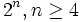$2^n, n \ge 4$, there are exactly three maximal class groups: dihedral, semidihedral, and generalized quaternion. For order 32, the groups are: dihedral group:D32, semidihedral group:SD32, and generalized quaternion group:Q32.

### Numbers of equivalence classes of groups

Equivalence relation on groups Number of equivalence classes of groups of order 32 Sizes of equivalence classes, i.e., number of isomorphism classes of groups within each equivalence class (should add up to 51) More information
isoclinic groups (i.e., Hall-Senior families) 8 7, 15, 10, 9, 2, 2, 3, 3 #Up to isoclinism, see also classification of groups of order 32
isologic groups with respect to nilpotency class two 3 33, 15, 3 #Up to isologism for class two. This is a coarser equivalence relation than being isoclinic.
isologic groups with respect to nilpotency class three 2 48, 3 #Up to isologism for class three. This is a coarser equivalence relation than being isologic with respect to nilpotency class two.
having the same conjugacy class size statistics 6 7,15,19,2,5,3 Element structure of groups of order 32#Conjugacy class sizes. Note that isoclinic groups of the same order have the same conjugacy class size statistics, so this is a coarser equivalence relation than being isoclinic.
having the same degrees of irreducible representations 6 7,15,19,2,5,3 See Linear representation theory of groups of order 32#Degrees of irreducible representations. Note that for order 32, the degrees of irreducible representations and the conjugacy class size statistics determine each other, but this breaks down for higher orders. Also, note that this is a coarser equivalence relation than being isoclinic.
1-isomorphic groups 38 1 (29 times), 2 (6 times), 3 (2 times), 4 (1 time) Element structure of groups of order 32#1-isomorphism

## The list

Note there's an ambiguity that makes the table below incomplete: the Hall-Senior numbers of groups with GAP IDs 13 and 14 are 29 and 30 (symbol$\Gamma_3d_1$ and$\Gamma_3d_2$ respectively) but it's not yet clear which GAP ID corresponds to which Hall-Senior number.

Group Second part of GAP ID (GAP ID is (32,second part)) Hall-Senior number (among groups of order 32) Hall-Senior symbol Nilpotency class Probability in cohomology tree probability distribution (proper fraction) Probability in cohomology tree probability distribution (as numerical value)
Cyclic group:Z32 1 7$(5)$ 1 1/16 0.0625
SmallGroup(32,2) 2 18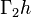$\Gamma_2h$ 2 59/2048 0.0288
Direct product of Z8 and Z4 3 5$(32)$ 1 51/1024 0.0498
Semidirect product of Z8 and Z4 of M-type 4 19$\Gamma_2i$ 2 49/1024 0.0479
SmallGroup(32,5) 5 20$\Gamma_2j_1$ 2 71/1024 0.0693
Faithful semidirect product of E8 and Z4 6 46$\Gamma_7a_1$ 3 13/1024 0.0127
SmallGroup(32,7) 7 47$\Gamma_7a_2$ 3 13/2048 0.0063
SmallGroup(32,8) 8 48$\Gamma_7a_3$ 3 13/2048 0.0063
SmallGroup(32,9) 9 27$\Gamma_3c_1$ 3 31/1024 0.0303
SmallGroup(32,10) 10 28$\Gamma_3c_2$ 3 37/1024 0.0361
Wreath product of Z4 and Z2 11 31$\Gamma_3e$ 3 13/512 0.0254
SmallGroup(32,12) 12 21$\Gamma_2j_2$ 2 45/512 0.0879
Semidirect product of Z8 and Z4 of semidihedral type 13 3 7/256 0.0273
Semidirect product of Z8 and Z4 of dihedral type 14 3 25/1024 0.0244
SmallGroup(32,15) 15 32$\Gamma_3f$ 3 1/32 0.0313
Direct product of Z16 and Z2 16 6$(41)$ 1 31/256 0.1211
M32 17 22$\Gamma_2k$ 2 15/256 0.0586
Dihedral group:D32 18 49$\Gamma_8a_1$ 4 3/1024 0.0029
Semidihedral group:SD32 19 50$\Gamma_8a_2$ 4 3/512 0.0059
Generalized quaternion group:Q32 20 51$\Gamma_8a_3$ 4 3/1024 0.0029
Direct product of Z4 and Z4 and Z2 21 3$(2^21)$ 1 637/65536 0.0097
Direct product of SmallGroup(16,3) and Z2 22 11$\Gamma_2c_1$ 2 695/65536 0.0106
Direct product of SmallGroup(16,4) and Z2 23 12$\Gamma_2c_2$ 2 349/16384 0.0213
SmallGroup(32,24) 24 16$\Gamma_2f$ 2 273/32768 0.0083
Direct product of D8 and Z4 25 14$\Gamma_2e_1$ 2 69/4096 0.0168
Direct product of Q8 and Z4 26 15$\Gamma_2e_2$ 2 123/16384 0.0075
SmallGroup(32,27) 27 33$\Gamma_4a_1$ 2 45/16384 0.0027
SmallGroup(32,28) 28 36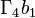$\Gamma_4b_1$ 2 33/4096 0.0081
SmallGroup(32,29) 29 37$\Gamma_4b_2$ 2 225/16384 0.0137
SmallGroup(32,30) 30 38$\Gamma_4c_1$ 2 129/16384 0.0079
SmallGroup(32,31) 31 39$\Gamma_4c_2$ 2 129/32768 0.0039
SmallGroup(32,32) 32 40$\Gamma_4c_3$ 2 111/16384 0.0068
SmallGroup(32,33) 33 41$\Gamma_4d$ 2 21/4096 0.0051
Generalized dihedral group for direct product of Z4 and Z4 34 34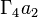$\Gamma_4a_2$ 2 45/65536 0.0007
SmallGroup(32,35) 35 35$\Gamma_4a_3$ 2 321/65536 0.0049
Direct product of Z8 and V4 36 4$(31^2)$ 1 543/16384 0.0331
Direct product of M16 and Z2 37 13$\Gamma_2d$ 2 637/16384 0.0389
Central product of D8 and Z8 38 17$\Gamma_2g$ 2 63/4096 0.0154
Direct product of D16 and Z2 39 23$\Gamma_3a_1$ 3 141/32768 0.0043
Direct product of SD16 and Z2 40 24$\Gamma_3a_2$ 3 237/16384 0.0145
Direct product of Q16 and Z2 41 25$\Gamma_3a_3$ 3 237/32768 0.0072
Central product of D16 and Z4 42 26$\Gamma_3b$ 3 45/8192 0.0055
Holomorph of Z8 43 44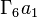$\Gamma_6a_1$ 3 45/8192 0.0055
SmallGroup(32,44) 44 45$\Gamma_6a_2$ 3 45/8192 0.0055
Direct product of E8 and Z4 45 2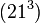$(21^3)$ 1 1023/1048576 0.0010
Direct product of D8 and V4 46 8$\Gamma_2a_1$ 2 825/1048576 0.0008
Direct product of Q8 and V4 47 9$\Gamma_2a_2$ 2 771/1048576 0.0007
Direct product of SmallGroup(16,13) and Z2 48 10$\Gamma_2b$ 2 329/262144 0.0013
Inner holomorph of D8 49 42$\Gamma_5a_1$ 2 35/131072 0.0003
Central product of D8 and Q8 50 43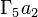$\Gamma_5a_2$ 2 21/131072 0.0002
Elementary abelian group:E32 51 1$(1^5)$ 1 1/1048576 0.0000

## Arithmetic functions

### Summary information

Here, the rows are arithmetic functions that take values between$0$ and$5$, and the columns give the possible values of these functions. The entry in each cell is the number of isomorphism classes of groups for which the row arithmetic function takes the column value. Note that all the row value sums must equal$51$. To view a list of all groups, click on the value in the cell and the list of all groups with GAP IDs appears.

Arithmetic function Value 0 Value 1 Value 2 Value 3 Value 4 Value 5 Mean (with equal weighting on all groups) Mean (with weighting by cohomology tree probability distribution)
prime-base logarithm of exponent 0 1 23 21 5 1 2.6471 3.1426
Frattini length 0 1 23 21 5 1 2.6471 3.1426
nilpotency class 0 7 26 15 3 0 2.2745 1.9889
derived length 0 7 44 0 0 0 1.8627 1.7228
minimum size of generating set (sometimes called rank, though it differs from rank of a p-group as used below) 0 1 19 24 6 1 2.7451 2.2039
rank of a p-group 0 2 21 23 4 1 2.6275 2.3064
normal rank of a p-group 0 4 23 19 4 1 2.5098 2.2431
characteristic rank of a p-group 0 7 26 14 3 1 2.3137 2.1972

## Families and classification

### Up to isoclinism

FACTS TO CHECK AGAINST for isoclinic groups (groups with an isoclinism between them):
by definition, isoclinic groups have isomorphic inner automorphism groups and isomorphic derived subgroups, with the isomorphisms compatible.
isoclinic groups have same nilpotency class|isoclinic groups have same derived length | isoclinic groups have same proportions of conjugacy class sizes | isoclinic groups have same proportions of degrees of irreducible representations

The information below collects groups based on the equivalence relation of being isoclinic groups. The equivalence classes are also called Hall-Senior families.

Family name Isomorphism class of inner automorphism group Isomorphism class of derived subgroup Number of members Nilpotency class Members Second part of GAP ID of members (sorted ascending) Hall-Senior numbers of members (sorted ascending)$\Gamma_1$ trivial group trivial group 7 1 all abelian groups of order 32: [SHOW MORE] 1,3,16,21,36,45,51 1-7$\Gamma_2$ Klein four-group cyclic group:Z2 15 2 [SHOW MORE] 2,4,5,12,17,22,23,24,25,26,37,38,46,47,48 8-22$\Gamma_3$ dihedral group:D8 cyclic group:Z4 10 3 [SHOW MORE] 9,10,11,13,14,15,39,40,41,42 23-32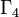$\Gamma_4$ elementary abelian group:E8 Klein four-group 9 2 [SHOW MORE] 27-35 33-41$\Gamma_5$ elementary abelian group:E16 cyclic group:Z2 2 2 inner holomorph of D8, central product of D8 and Q8 49, 50 42, 43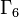$\Gamma_6$ direct product of D8 and Z2 cyclic group:Z4 2 3 holomorph of Z8, SmallGroup(32,44) 43,44 44,45$\Gamma_7$ SmallGroup(16,3) Klein four-group 3 3 [SHOW MORE] 6-8 46-48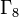$\Gamma_8$ dihedral group:D16 cyclic group:Z8 3 4 [SHOW MORE] 18-20 49-51
Total (8 rows) -- -- 51 -- -- -- --

### Up to Hall-Senior genus

Genus name Members Second part of GAP ID of members Hall-Senior numbers of members$(1^5)$ elementary abelian group:E32 51 1$(21^3)$ direct product of E8 and Z4 45 2$(2^21)$ direct product of Z4 and Z4 and Z2 21 3$(31^2)$ direct product of Z8 and V4 36 4$(32)$ direct product of Z8 and Z4 3 5$(41)$ direct product of Z16 and Z2 16 6$(5)$ cyclic group:Z32 1 7$\Gamma_2a$ direct product of D8 and V4, direct product of Q8 and V4 46,47 8,9$\Gamma_2b$ direct product of SmallGroup(16,13) and Z2 48 10$\Gamma_2c$ direct product of SmallGroup(16,3) and Z2, direct product of SmallGroup(16,4) and Z2 22,23 11,12$\Gamma_2d$ direct product of M16 and Z2 37 13$\Gamma_2e$ direct product of D8 and Z4, direct product of Q8 and Z4 25, 26 14, 15$\Gamma_2f$ SmallGroup(32,24) 24 16$\Gamma_2g$ central product of D8 and Z8 38 17$\Gamma_2h$ SmallGroup(32,2) 2 18$\Gamma_2i$ semidirect product of Z8 and Z4 of M-type 4 19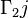$\Gamma_2j$ SmallGroup(32,5), SmallGroup(32,12) 5, 12 20, 21$\Gamma_2k$ M32 17 22$\Gamma_3a$ direct product of D16 and Z2, direct product of SD16 and Z2, direct product of Q16 and Z2 39,40,41 23,24,25$\Gamma_3b$ central product of D8 and Z8 42 26$\Gamma_3c$ SmallGroup(32,9), SmallGroup(32,10) 9,10 27,28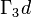$\Gamma_3d$ semidirect product of Z8 and Z4 of semidihedral type, semidirect product of Z8 and Z4 of dihedral type 13,14 29,30$\Gamma_3e$ wreath product of Z4 and Z2 11 31$\Gamma_3f$ SmallGroup(32,15) 15 32$\Gamma_4a$ SmallGroup(32,27), generalized dihedral group for direct product of Z4 and Z4, SmallGroup(32,35) 27, 34, 35 33, 34, 35$\Gamma_4b$ SmallGroup(32,28), SmallGroup(32,29) 28,29 36,37$\Gamma_4c$ SmallGroup(32,30), SmallGroup(32,31), SmallGroup(32,32) 30,31,32 38,39,40$\Gamma_4d$ SmallGroup(32,33) 33 41$\Gamma_5a$ inner holomorph of D8, central product of D8 and Q8 49, 50 42, 43$\Gamma_6a$ holomorph of Z8, SmallGroup(32,44) 43, 44 44, 45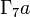$\Gamma_7a$ faithful semidirect product of E8 and Z4, SmallGroup(32,7), SmallGroup(32,8) 6, 7, 8 46, 47, 48$\Gamma_8a$ dihedral group:D32, semidihedral group:SD32, generalized quaternion group:Q32 18, 19, 20 49, 50 51

### Up to isologism for class two

Under the equivalence relation of being isologic groups with respect to the variety of groups of nilpotency class two, the equivalence classes are as follows (the table is incomplete):

Isomorphism class of quotient by second center Isomorphism class of third member of lower central series Number of groups Nilpotency class(es) Second part of GAP ID of members (sorted ascending) Hall-Senior numbers of members (sorted ascending) Smallest order of group isologic to these groups Stem groups (groups of smallest order)
trivial group trivial group 33 1,2 1-5,12,16,17,21-38,45-51 1-22,33-43 1 trivial group
Klein four-group cyclic group:Z2 15 3 6-11, 13-15, 39-44 23-32, 44-48 16 dihedral group:D16, semidihedral group:SD16, generalized quaternion group:Q16
dihedral group:D8 cyclic group:Z4 3 4 18-20 49-51 32 dihedral group:D32, semidihedral group:SD32, generalized quaternion group:Q32
-- (3 rows) -- 51 -- -- -- -- --

### Up to isologism for class three

Under the equivalence relation of being isologic groups with respect to the variety of groups of class at most three, there are two equivalence classes:

Isomorphism class of quotient by third center Isomorphism class of fourth member of lower central series Number of groups Nilpotency class(es) Second part of GAP ID of members (sorted ascending) Hall-Senior numbers of members (sorted ascending) Smallest order of group isologic to these groups Stem groups (groups of smallest order)
trivial group trivial group 48 1,2,3 1-17,21-51 1-48 1 trivial group
Klein four-group cyclic group:Z2 3 4 18-20 49-51 32 dihedral group:D32, semidihedral group:SD32, generalized quaternion group:Q32
-- (2 rows) -- 51 -- -- -- -- --

### Up to isologism for higher class

For class four or higher, all groups of order 32 are isologic to each other.

## Element structure

Further information: element structure of groups of order 32

## Subgroup structure

Further information: subgroup structure of groups of order 32

## Linear representation theory

Further information: linear representation theory of groups of order 32